# RD Sharma Solutions for Class 8 Math Chapter 6 - Algebraic Expressions and Identities (Part-3) Notes | Study RD Sharma Solutions for Class 8 Mathematics - Class 8

## Class 8: RD Sharma Solutions for Class 8 Math Chapter 6 - Algebraic Expressions and Identities (Part-3) Notes | Study RD Sharma Solutions for Class 8 Mathematics - Class 8

The document RD Sharma Solutions for Class 8 Math Chapter 6 - Algebraic Expressions and Identities (Part-3) Notes | Study RD Sharma Solutions for Class 8 Mathematics - Class 8 is a part of the Class 8 Course RD Sharma Solutions for Class 8 Mathematics.
All you need of Class 8 at this link: Class 8

#### Answer 5: To find the product, we will use distributive law as follows:

6x/5 (x3+y3)

=6x/5×x3+6x/5×y3

=6/5×(x×x3)+6/5×(x×y3)

=6/5×(x1+3)+6/5×(x×y3)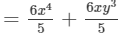Thus, the answer is.

#### Question 8: Find the following product: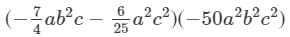#### Answer 8: To find the product, we will use distributive law as follows: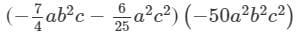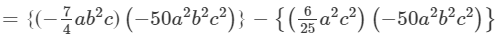={{−7/4×(−50)}(a×a2)×(b2×b2)×(c×c2)}−{(625)(−50)(a2×a2)×(b2)×(c2×c2)}

={7/4×(50)}(a1+2b2+2c1+2){(6/25)(50)(a2+2b2c2+2)}

=175/2 a3b4c3(12a4b2c4)

=175/2 a3b4c3+12a4b2c4

Thus, the answer is 175/2 a3b4c3+12a4b2c41752a3b4c3+12a4b2c4.

#### Question 9: Find the following product: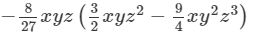#### Answer 9: To find the product, we will use the distributive law in the following way: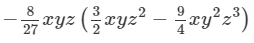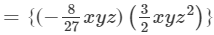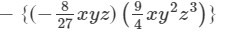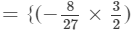(x×x)×(y×y)×(z×z2)}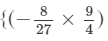(x×x)×(y×y2)×(z×z3)}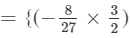(x1+1y1+1z1+2)}−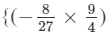(x1+1y1+2z1+3)}

23y+7=-11y2×3y+-11y2×7=-11×3y2×y+-11×7×y2=-33y2+1+-77×y2=-33y3-77y2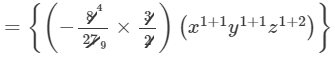-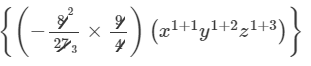=4/9 x2y2z3+2/3 x2y3z4

Thus, the answer is 4/9 x2y2z3+2/3 x2y3z4

#### Question 10: Find the following product: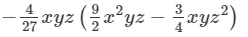#### Answer 10: To find the product, we will use distributive law as follows: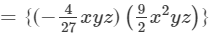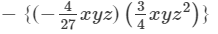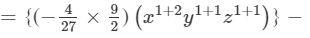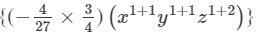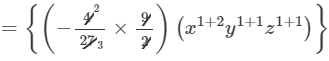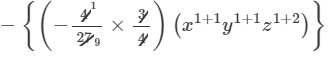=2/3 x3y2z2+1/9 x2y2z3

Thus, the answer is 2/3 x3y2z2+1/9 x2y2z3

#### Question 13: Find the following product:

250.5xy (xz+y/10)xz+y10

#### Question 14: Find the following product: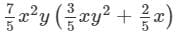#### Answer 14: To find the product, we will use distributive law as follows: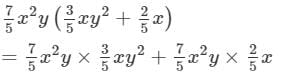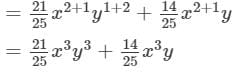Thus, the answer is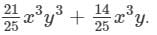#### Question 15: Find the following product:

4/3 a(a2 + b2  3c2)43a(a2 + b2 - 3c2)

#### Answer 15: To find the product, we will use distributive law as follows:

4/3 a(a2+b23c2)=4/3 a×a2+4/3 a×b24/3 a×3c2=4/3 a1+2+4/3 ab24ac2=4/3 a3+4/3 ab24ac2

Thus, the answer is 4/3 a3+4/3 ab24ac2

#### Answer 16: To find the product, we will use distributive law as follows:24x2(1−2x)=24x2×1−24x2×2x=24x2−48x1+2=24x2−48x324x21-2x=24x2×1-24x2×2x=24x2-48x1+2=24x2-48x3Substituting  x = 3 in the result, we get:

24x248x3=24(3)248(3)3=24×948×27=2161296=1080 Thus, the product is (24x248x3) and its value for x = 3 is (1080)(24x2-48x3) and its value for x = 3 is (-1080).

#### Answer 17: To find the product, we will use distributive law as follows:−3y(xy+y2)=−3y×xy+(−3y)×y2=−3xy1+1−3y1+2=−3xy2−3y3Substituting x = 4 and y = 5 in the result, we get:

3xy23y3=3(4)(5)23(5)3=3(4)(25)3(125)=300375=675 Thus, the product is (3xy23y3-3xy2-3y3), and its value for x = 4 and y = 5 is (-675).

#### Answer 18: To find the product, we will use distributive law as follows:

3/2 x2y3×(2xy)=(3/2 x2y3×2x)(3/2 x2y3×y)=(3x2+1y3)(3/2 x2y3+1)=3x3y3+3/2 x2y4

Substituting x = 1 and y = 2 in the result, we get:

3x3y3+3/2 x2y4=3(1)3(2)3+3/2 (1)2(2)4=3×1×8+3/2 ×1×16=24+24=0Thus, the product is 3x3y3+3/2 x2y4-3x3y3+32x2y4, and its value for x = 1 and y = 2 is 0.